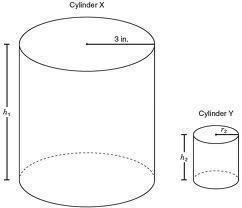# Cubic Capacity

The cubic capacity refers to a volume. Most of the time, it connotes to a cylinder head’s volume. The measurement is applied when the piston is at the lower dead center. However, the term can also be applied in other ways.

How to Determine a Cylinder’s Capacity

This method is used to evaluate the volume of hollow cylinders. You’ll need an empty can, measuring tape and calculator.

The formula is very simple. It is the end area which you multiply by the cylinder’s height. The end of a cylinder is a circle. All you have to do is measure the cylinder’s height. Use the tape measure. The next step is to determine the cylinder’s circumference. You can get the figure by wrapping the tape around the cylinder.

To calculate the radius, divide the circumference by pi (3.1416). A circumference of 18.85 cm will have a 6 cm diameter. The radius is 3 cm or half of the diameter. Assuming the height is 20 cm, the volume will be 578.232 cubic cm.

How to Get Cubic Feet in a Propane Tank

When studying information about cubic capacity, you may find the need to calculate cubic feet in other cylinder-like objects. The following method will work for propane tanks, barrels and oil storage tanks. As in the previous exercise, a tape measure and calculator will be needed.

Step 1

Use the tape measure to get the distance from the cylinder ends. Measure at the widest points. The results should be in inches.Step 2

To obtain the propane tank’s radius, divide the diameter in exactly half. If the tank’s diameter is 12 inches, you will get a 6 inch radius. Next, square the radius figure. For instance, 6 x 6 = 36.

Step 3

Use your calculator and multiply the square radius by 3.14. In this example, 36 x 3.14 = 113.04 square inches.

Step 4

Use your tape measure to get the cylinder length. Multiply the number by the figure you got in the previous step. Assume the cylinder length is 24 inches. The process would be 24 x 113.04 = 2712.96 square inches.

Step 5

The last step is to divide the cylinder’s square inch volume by 1728. The result is the cubic feet. In this case, the answer is 1.57 cubic feet.

Trying to figure out the cubic capacity of various objects is not that difficult to do. Even a simple calculator will be able to supply you with the proper figures.